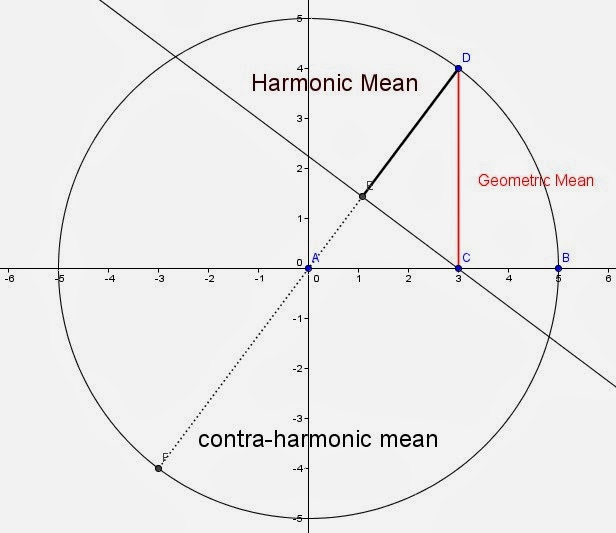## Thursday, 20 February 2014

### Harmonic Geometry, Some Nice Problems about the Harmonic Mean

The problem above is often called the crossed ladders problem, as it is on the Wikipedia site where I found this image. The idea is that the two ladders are each resting against the foot of a building and leaning against the opposite building. Given the heights A and B at which they touch the opposite buildings, the challenge is to find the height, h at which they cross.

I had recently planned a talk on a collection of harmonic mean problems, particularly some geometric ones, but it was cancelled due to weather, so I thought I would point out some of the interesting geometric problems that are related to the harmonic mean in this blog.

For the student/teacher/reader who is not familiar with the Harmonic mean, a couple of notes to lay the foundation seem appropriate.

The harmonic mean is one of the classic old Greek means called Pythagorean means because they were, supposedly, known to the Pythagoras or his followers. The harmonic mean is associated with the musical interval known as the Perfect fourth. An octave change upwards in pitch reflects a doubling of the wavelength frequency (a 1:2 ratio), the harmonic mean of 1 and 2 is 4/3.
The actual definition of the harmonic mean usually sounds convoluted to students. The harmonic mean is the reciprocal of the average of the reciprocals of the values. The algebraic notation is only a little clearer. For a set of numbers xi the harmonic mean, H, is given by $H = \frac{n}{ \frac{1}{x_1} + \frac {1}{x_2} + \dotsb+ \frac {1}{x_n} }$

Fortunately for two values a and b, the whole thing simplifies down to $\frac{2ab}{a+b}$.The harmonic mean is often shown geometrically in a trapezoid resembling the crossed ladder problem. The harmonic mean is given by the red line, and the solution to the crossed ladders problem is 1/2  the harmonic means of the wall heights.

Another classic geometry illustration  is used to show the relationship between the means of two values in a circle.  When the diameter is divided into two parts, a and b, then the relation between the Arithmetic mean which equals the length of the radius, the Geometric mean and Harmonic mean will always be related by  $A \ge G \ge H$A slightly different version shows the contra -harmonic mean which is equal to the length of the diameter less the harmonic mean.    The contr-harmonic mean is the ratio of the sum of the squares divided by the sum of the values, or $\frac{x_1^2 + x_2^2 + \dotsb + x_n^2}{x_1 + x_2 + \dotsb + x_n}$

In the trapezoidal view of the harmonic mean of two numbers, the arithmetic mean (green) joins the midpoints of the two non-parallel sides and the contra-harmonic mean (blue) is the same distance away from the arithmetic mean as the harmonic mean.

So with that bit of information let's talk about a few problems.  I will give all the problems here, and provide solutions in a second blog: The key in all of them is not just a solution, but a solution related to the harmonic mean.  (many are more easily solved w/o using harmonic mean)

a square inscribed in a right triangle whose legs are A ft and B feet (with one corner of the square at the right angle of the triangle), what is the length of a side of the square?

A 3-d version of the same problem may be more of a challenge for the more talented students:
A cube inscribed in a right rectangular tetrahedron with legs of a, b, and c has a cube inscribed with one vertex at (0,0,0).

What is the length of an edge of the cube?

Another with a triangle,
Given a 5-12-13 Right Triangle, Find a relation between the radius of the inscribed circle and the altitudes.   Is this property true of a non-right triangle?

For the student of the conics, In the ellipse $9x^2+4y^2=36$, find the length of the semi-latus rectum.

Hopefully I still remember how to solve all these, and will try to post a blog with at least one explanation for each, but would love to hear your solutions, and additional geometric problems involving the harmonic mean.
Enjoy.

The solutions (I hope) are now posted here.

Addendum: Recently came across this neat one from Antonio Gutierrez@gogeometry.  Note the radii of the two Archimedean Twins.Anonymous said...

A really interesting post thanks. Lots of ideas for me to draw upon in this year's teaching as I have a particularly gifted group of 15 year olds. Thanks for taking the time to write this.
Rob

Dave Giles said...

You may want to check the formula you have given for H.

Unknown said...

Agree with Dave Giles. H should be 1/H I think...

Pat's Blog said...

Thanks Eric and Simon, I have trouble getting Blogger to accept complex fractions so I end up trying to alter them.... Maybe My LaTex needs work.